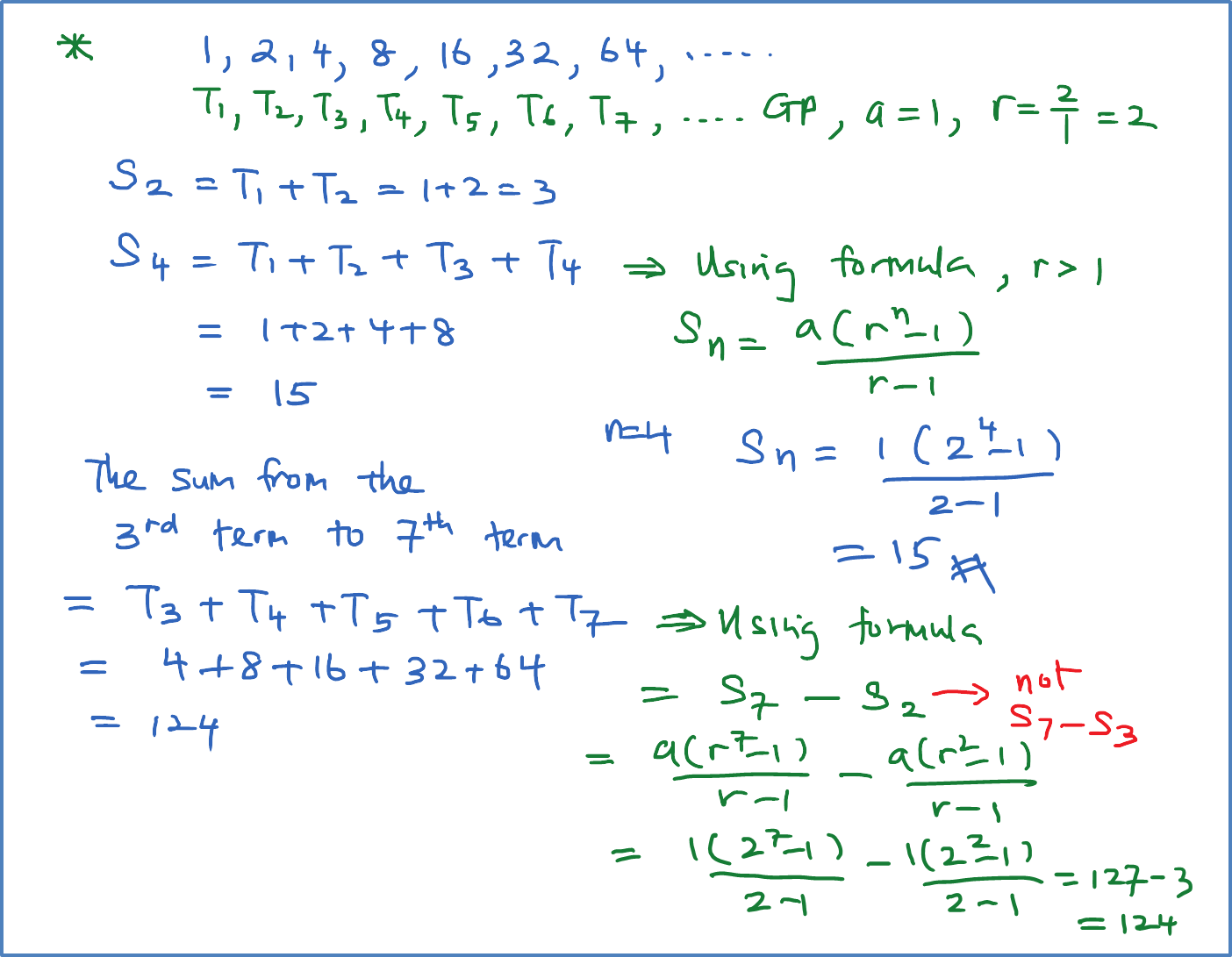\

# 5.4.3 Sum of the First n Terms of a Geometric Progression

5.4.3 Sum of the First n Terms of Geometric Progressions

(F) Sum of the First Terms of Geometric Progressions
$\overline{)\begin{array}{l}\text{}{S}_{n}=\frac{a\left({r}^{n}-1\right)}{r-1},\text{}r>1\text{}\\ \text{}{S}_{n}=\frac{a\left(1-{r}^{n}\right)}{1-r},\text{}r<1\end{array}}$ a = first term
r = common ratio
n = number of term
Sn = sum of the first n terms

Example 1:
Find the sum of each of the following geometric progressions.
(a) 1, 2, 4, …  up to the first 7 terms
(b) 9, 3,   1,   ,   …    up to the first 6 terms
(c) 12, 3, …., $\frac{3}{64}$ [Smart TIPS: You can find n if you know the last term]

Solution:### 1 thought on “5.4.3 Sum of the First n Terms of a Geometric Progression”

1.can i get the next link for sum to infinty of geo progressions